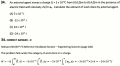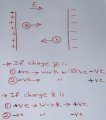# work done by an external force in moving a charge Q in an electric field

#### skyline977

Joined Jan 14, 2021
29
I am trying to understand the reason for having the work to be negative, the question and answer are as follows:However, the work done should be positive because the charge is positive and thus it accelerates in the same direction of the electric field. This means the angle between them = 0 and because it's a dot product it's cosine the angle between the electric field and the charge movement which is +1. If the charge is negative then it would accelerate in the opposite direction making a 180 degrees, and cosine will be -1 and so will the work become also negative Right?

#### BobTPH

Joined Jun 5, 2013
6,514
The sign of the work depends on whether the charge has gained or lost energy in the move. If it gains energy, the work is positive, if it loses, the work is negative. For a move from lower to higher potential, the sign of the work is the same as that of the charge. For a move to a lower potential, it is is opposite.
Bob

•skyline977

#### skyline977

Joined Jan 14, 2021
29
The sign of the work depends on whether the charge has gained or lost energy in the move. If it gains energy, the work is positive, if it loses, the work is negative. For a move from lower to higher potential, the sign of the work is the same as that of the charge. For a move to a lower potential, it is is opposite.
Bob
So, if I understand you correctly I simplified it in the following sketch please review it if it's correct or not.Thanks

#### BobTPH

Joined Jun 5, 2013
6,514
Yep.

Bob

•skyline977